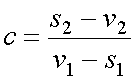## Coefficient of Restitution

The coefficient of restitution (denoted by the symbol c in our formulas) is a measure of the elasticity of the collision between ball and racquet. Elasticity is a measure of how much bounce there is, or in other words, how much of the kinetic energy of the colliding objects before the collision remains as kinetic energy of the objects after the collision. With an inelastic collision, some kinetic energy is transformed into deformation of the material, heat, sound, and other forms of energy, and is therefore unavailable for use in moving.

A perfectly elastic collision has a coefficient of restitution of 1. Example: two diamonds bouncing off each other. A perfectly plastic, or inelastic, collision has c = 0. Example: two lumps of clay that don’t bounce at all, but stick together. So the coefficient of restitution will always be between zero and one.

The coefficient of restitution is the ratio of the differences in velocities before and after the collision. In other words, the difference in the velocities of the two colliding objects after the collision, divided by the difference in their velocities before the collision. In symbolic language:c = coefficient of restitution

v1 = linear velocity of the racquet mass center before impact

s1 = linear velocity of the ball before impact (will be negative according to our convention that away from the player is positive)

v2 = linear velocity of the racquet mass center after impact

s2 = linear velocity of the ball after impact

From the coefficient of restitution formula, it follows that

s2 - v2 = c(v1 - s1)

To find the coefficient of restitution in the case of a falling object bouncing off the floor, or off a racquet on the floor, use the following formula:c = coefficient of restitution (dimensionless)

h = bounce height

H = drop height

For the Benchmark Conditions, the coefficient of restitution used is 0.85 for all racquets, eliminating the variables of string tension and frame stiffness which could add or subtract from the coefficient of restitution.